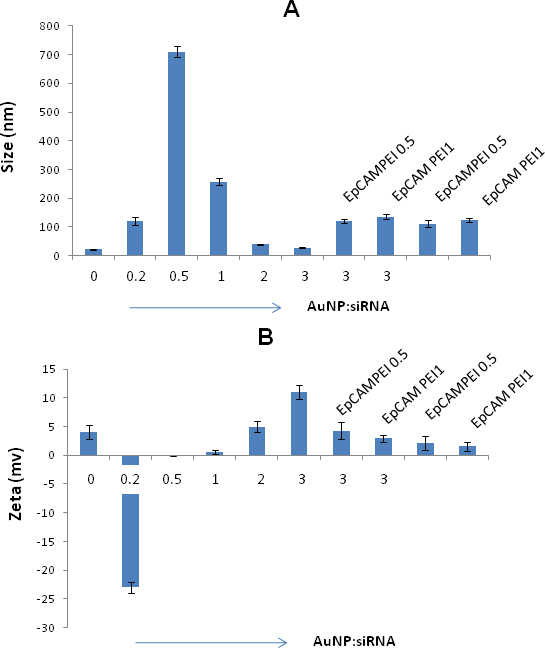Figure 3 of Mitra, Mol Vis 2013; 19:1029-1038.

Figure 3. Determination of Size and charge of nanoconjugates. The size distribution of AuNPs mixed with siRNA and/or conjugated EpCAM antibody was determined at varying AuNP:siRNA ratios. A: The siRNA conjugated gold nanoparticles showed increased size of about 710 nm and 257 nm at the ratios of 0.5 and 1, respectively. The optimal size of about 28 nm was achieved at the AuNP:siRNA weight ratio of 3:1. The size of the gold nanoparticles conjugated with PEI and EpCAM antibody at a ratio of 0.5 and 1 was 111 and 124, respectively. B: The zeta potential measurements of the AuNPs mixed with siRNA and/or conjugated EpCAM antibody. The zeta potential was almost neutral at the weight ratios of 0.5 and 1, which suggests particle aggregation in the solution. The zeta potential was shifted to 5 and 11 at the weight ratios of 2 and 3, respectively. The zeta potential was 2.1 and 1.6 at EpCAMPEI ratio of 0.5 and 1, respectively. The optimal size of about 28 nm was achieved at the AuNP:siRNA weight ratio of 3:1. The size of the AuNP-PEI-EpAb at a ratio of 0.5 and 1 was 111 and 124, respectively. B: The zeta potential measurements of the AuNPs mixed with siRNA and/or conjugated EpCAM antibody. The zeta potential was almost neutral at the weight ratios of 0.5 and 1, which suggests particle aggregation in the solution. The zeta potential was shifted to 5 and 11 at the weight ratios of 2 and 3, respectively. The zeta potential was 2.1 and 1.6 at EpCAM-PEI 0.5 and 1, respectively.